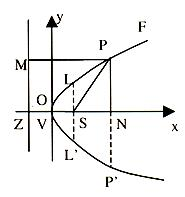×#### Thank you for registering.

One of our academic counsellors will contact you within 1 working day.

Click to Chat

1800-1023-196

+91-120-4616500

CART 0

• 0

MY CART (5)

Use Coupon: CART20 and get 20% off on all online Study Material

ITEM
DETAILS
MRP
DISCOUNT
FINAL PRICE
Total Price: Rs.

There are no items in this cart.
Continue Shopping```Introduction to ParabolaConics or conic sections are the curves corresponding to various plane sections of a right circular cone by cutting that cone in different ways.

Each point lying on these curves satisfies a special condition, which actually leads us towards the mathematical definition of conic sections.

If a point moves in plane in such a way that the ratio of its distance from a fixed point to its perpendicular distance from a fixed straight line, always remains constant, then the locus of that point I called a Conic Section.
The fixed point is called the focus and the fixed line is called directrix of the conic. The constant ratio is called the eccentricity and is denoted by e.
According to the value of there are three types o conic i.e. for e = 1, e < 1 and e > 1 the corresponding conic is called parabola, ellipse and hyperbola respectively.
A conic section or conic is the locus of a point, which moves so that its distance from a fixed point is in a constant ratio to its distance from a fixed straight line, not passing through the fixed point.
The fixed point is called the focus.
• The fixed straight line is called the directrix.
• The constant ratio is called the eccentricity and is denoted by e.
• When the eccentricity is unity i.e. e = 1, the conic is called a Parabola; when e < 1, the conic is called an ellipse; and when e > 1, the conic is called a hyperbola.
• The straight line passing through the focus and perpendicular to the directrix is called the axis of the parabola.
• The point of intersection of a conic with its axis is called Vertex.
• The chord passing through focus and perpendicular to axis is called Latus Rectum.
• Any chord of the parabola which is perpendicular to the axis is called double ordinate.
• The straight line perpendicular to axis of the parabola passing through vertex is called tangent at the vertex.

Axis of the conic

The line through focus and perpendicular to the directrix is called the axis of the conic. The intersection point o conic with axis is known as the vertex of the conic.
Enquiry: How do we mathematically define a parabola and what are its various features?
The locus of the point, which moves such that its distance from a fixed point (i.e. focus) is always equal to its distance from a fixed straight line (i.e. directrix), is called Parabola.

Equation of Parabola

Let S be the focus, V be the vertex, ZM be the directrix and x-axis be the axis of parabola. We require therefore the locus of a point P, whichmoves so that its distance from S, is always equal to PM i.e. its perpendicular distance from ZM. After appropriate configuration let S = (a, 0)

We have ten SP2 = PM2

⇒ (x – a)2 + y2 = (a + x)2

⇒ y2 = 4ax This is the standard equation of a parabola.

To read more, Buy study materials of Parabola comprising study notes, revision notes, video lectures, previous year solved questions etc. Also browse for more study materials on Mathematics here.

```### Course Features

• 731 Video Lectures
• Revision Notes
• Previous Year Papers
• Mind Map
• Study Planner
• NCERT Solutions
• Discussion Forum
• Test paper with Video Solution